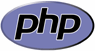# PHP: Calculate Real Differences Between Two Dates or TimestampsI was using simple function to calculate difference between two dates and timestamps until I noticed, it’s not working correctly in long intervals. It’s very easy to calculate difference between two timestamps in seconds, but it’s much more complicated print difference in human readable format. The Internet can be found in a wide range of ways to do this thing, but as a rule they use a fixed amount of seconds for the year and the month. So if we calculate year with using 365 or 365.25 days and month using 30 or 31 then the difference is not accurate, because of leap years, DST (Daylight Saving Time) and so on.

Because of this problem, I decided to make a function (at least in the short testing) to return the right kind of differences between the UNIX timestamps and dates in human readable format. This function uses PHP strtotime function to calculate real differences and can handle leap years and DST. This function can also return Twitter like about texts with precision parameter.

## PHP dateDiff function for calculating real differences between dates and UNIX timestamps

``````
<?php

// Set timezone
date_default_timezone_set("UTC");

// Time format is UNIX timestamp or
// PHP strtotime compatible strings
function dateDiff(\$time1, \$time2, \$precision = 6) {
// If not numeric then convert texts to unix timestamps
if (!is_int(\$time1)) {
\$time1 = strtotime(\$time1);
}
if (!is_int(\$time2)) {
\$time2 = strtotime(\$time2);
}

// If time1 is bigger than time2
// Then swap time1 and time2
if (\$time1 > \$time2) {
\$ttime = \$time1;
\$time1 = \$time2;
\$time2 = \$ttime;
}

// Set up intervals and diffs arrays
\$intervals = array('year','month','day','hour','minute','second');
\$diffs = array();

// Loop thru all intervals
foreach (\$intervals as \$interval) {
// Create temp time from time1 and interval
\$ttime = strtotime('+1 ' . \$interval, \$time1);
// Set initial values
\$looped = 0;
// Loop until temp time is smaller than time2
while (\$time2 >= \$ttime) {
// Create new temp time from time1 and interval
\$ttime = strtotime("+" . \$add . " " . \$interval, \$time1);
\$looped++;
}

\$time1 = strtotime("+" . \$looped . " " . \$interval, \$time1);
\$diffs[\$interval] = \$looped;
}

\$count = 0;
\$times = array();
// Loop thru all diffs
foreach (\$diffs as \$interval => \$value) {
// Break if we have needed precission
if (\$count >= \$precision) {
break;
}
// if value is bigger than 0
if (\$value > 0) {
// Add s if value is not 1
if (\$value != 1) {
\$interval .= "s";
}
// Add value and interval to times array
\$times[] = \$value . " " . \$interval;
\$count++;
}
}

// Return string with times
return implode(", ", \$times);
}

?>
``````

## dateDiff function example usage

strtotime examples:

``````
echo dateDiff("2010-01-26", "2004-01-26") . "\n";
echo dateDiff("2006-04-12 12:30:00", "1987-04-12 12:30:01") . "\n";
echo dateDiff("now", "now +2 months") . "\n";
echo dateDiff("now", "now -6 year -2 months -10 days") . "\n";
echo dateDiff("2009-01-26", "2004-01-26 15:38:11") . "\n";
``````

Output:

```6 years
18 years, 11 months, 30 days, 23 hours, 59 minutes, 59 seconds
2 months
6 years, 2 months, 10 days
4 years, 11 months, 30 days, 8 hours, 21 minutes, 49 seconds
```

UNIX timestamp and precision examples

``````
echo dateDiff(time(), time()-1000000, 1) . "\n";
echo dateDiff(time(), time()-1000000, 3) . "\n";
echo dateDiff(time(), time()-1000000, 6) . "\n";
``````

Output:

```11 days
11 days, 13 hours, 46 minutes
11 days, 13 hours, 46 minutes, 40 seconds
```

Converting text format back to UNIX timestamp example

``````
\$time1 = time();
\$time2 = \$time1-10000000;
echo \$diff = dateDiff(\$time1, \$time2) . "\n";
echo \$time1 . "\n";
echo strtotime(" +".\$diff, \$time2) . "\n";
``````

Output:

```3 months, 23 days, 17 hours, 46 minutes, 40 seconds
1264514564
1264514564
```

## 226 comments on “PHP: Calculate Real Differences Between Two Dates or Timestamps”

This site uses Akismet to reduce spam. Learn how your comment data is processed.

Close